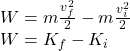Find the net work W done on the particle by the external forces during the motion of the particle in terms of the initial and final kinetic

Question

Find the net work W done on the particle by the external forces during the motion of the particle in terms of the initial and final kinetic energies.Express your answer in terms of Kinitial and Kfinal.W=

in progress 0
5 months 2021-09-03T06:26:36+00:00 1 Answers 9 views 0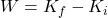Explanation:

The work done on a particle by external forces is defined as: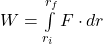According to Newton’s second law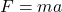. Thus: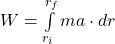Acceleration is defined as the derivative of the speed with respect to time: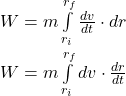Speed is defined as the derivative of the position with respect to time: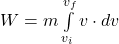Kinetic energy is defined as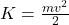: